# Refractometry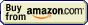## Refractometry » Critical angle

Critical angle is the incident angle at which light - instead of getting to the other side of phase boundary - gets refracted in such a way that it becomes parallel to the phase boundary surface. For smaller incident angles rays get through the boundary, for larger they get reflected back.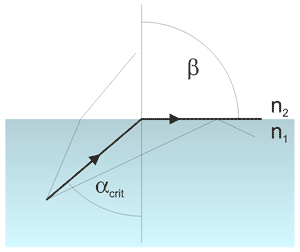Critical angle.

Critical angle can be easily calculated if we know refractive indices of both media. We start with the Snell's law: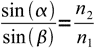1

α is the incident angle, β is the refracted angle. When refracted light gets paralell to the surface, refraction angle is 90°. That means sin(β) is 1 and critical angle can be found from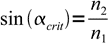2

Construction of most refractometers is based on the fact that critical angle is very easy to detect - instead of measuring the angle at which light gets refracted, we just measure angle at which boundary between light and dark appears.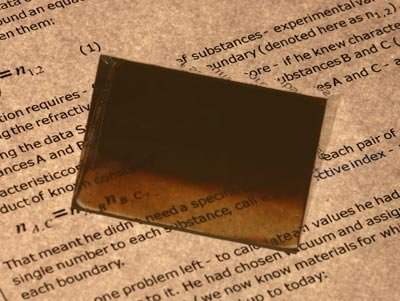Critical angle observed through a prism as a border between bright and dark area.

Critical angle observed in a cheap plastic prism. Paper lies on the glass and light shines from the bottom. Text is visible only when the light can pass through the prism. Light can enter the prism at different angles, and when it enters the prism, it is refracted. Light that is parallel to the prism surface gets refracted at the critical angle. That's exactly how the Abbé refractometer works. Prism is far from being perfect (its surfaces are not exactly flat), but the principle is well visible.

This site is in construction. More to come. Page was last modified on March 16 2011, 23:59:59.